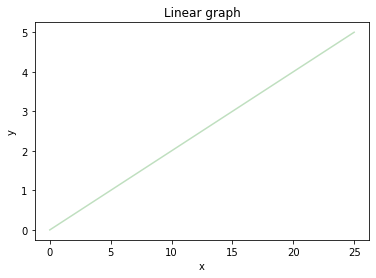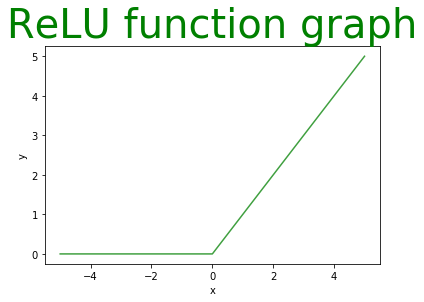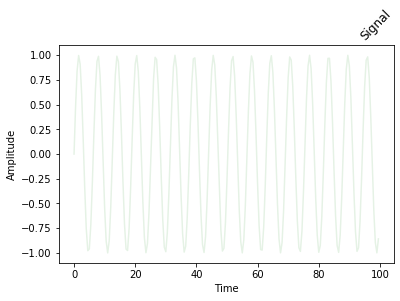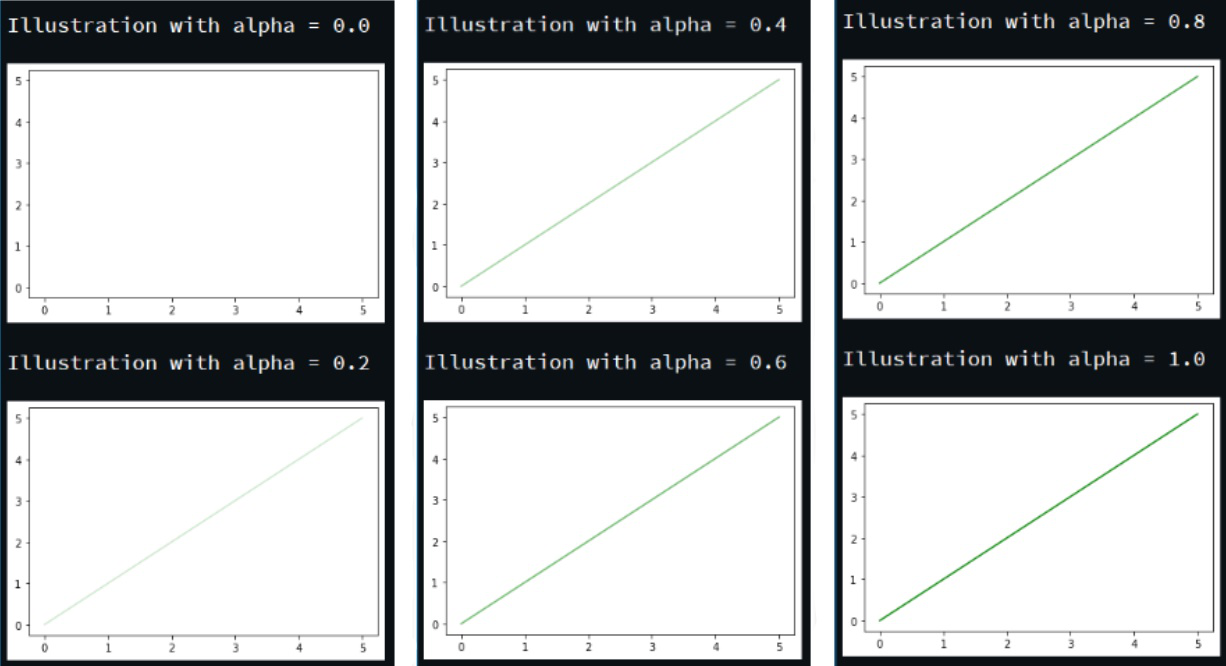GeeksforGeeks App
Open AppBrowser
Continue

# How to Change the Transparency of a Graph Plot in Matplotlib with Python?

Matplotlib is a library in Python and it is numerical — mathematical extension for NumPy library. Pyplot is a state-based interface to a matplotlib module which provides a MATLAB-like interface. There are various plots that can be used in Pyplot are Line Plot, Contour, Histogram, Scatter, 3D Plot, etc.

In order to change the transparency of a graph plot in matplotlib we will use the matplotlib.pyplot.plot() function. The plot() function in pyplot module of matplotlib library is used to make 2D illustrations.

Syntax: matplotlib.pyplot.plot(\*args, scalex=True, scaley=True, data=None, \*\*kwargs)

Parameters: This method accept the following parameters that are described below:

• x, y: These parameter are the horizontal and vertical coordinates of the data points. x values are optional.
• fmt: This parameter is an optional parameter and it contains the string value.
• data: This parameter is an optional parameter and it is an object with labelled data.

Returns: This returns the following:

• lines : This returns the list of Line2D objects representing the plotted data.

Another argument that we are going to use is alpha argument, this argument is responsible for the transparency of any illustration depicted using matplotlib library. Its value ranges from 0 to 1, by default its value is 1 representing the opaqueness of the illustration.

Example 1:

## Python3

 `# importing module``import` `matplotlib.pyplot as plt`` ` `# assigning x and y coordinates``y ``=` `[``0``, ``1``, ``2``, ``3``, ``4``, ``5``]``x ``=` `[``0``, ``5``, ``10``, ``15``, ``20``, ``25``]`` ` `# depicting the visualization``plt.plot(x, y, color``=``'green'``, alpha``=``0.25``)``plt.xlabel(``'x'``)``plt.ylabel(``'y'``)`` ` `# displaying the title``plt.title(``"Linear graph"``)`` ` `plt.show()`

Output:In the above program, the linear graph is depicted with transparency i.e alpha=0.25.

Example 2:

## Python3

 `# importing module``import` `matplotlib.pyplot as plt`` ` ` ` `# assigning x and y coordinates``x ``=` `[``-``5``, ``-``4``, ``-``3``, ``-``2``, ``-``1``, ``0``, ``1``, ``2``, ``3``, ``4``, ``5``]``y ``=` `[]`` ` `for` `i ``in` `range``(``len``(x)):``    ``y.append(``max``(``0``, x[i]))`` ` `# depicting the visualization``plt.plot(x, y, color``=``'green'``, alpha``=``0.75``)``plt.xlabel(``'x'``)``plt.ylabel(``'y'``)`` ` `# displaying the title``plt.title(label``=``"ReLU function graph"``,``                ``fontsize``=``40``,``                ``color``=``"green"``)`

Output:Here, the plot is quite opaque as the alphavalue is close to 1(opaque).

Example 3:

## Python3

 `# importing modules``from` `matplotlib ``import` `pyplot``import` `numpy`` ` `# assigning time values of the signal``# initial time period, final time period``# and phase angle``signalTime ``=` `numpy.arange(``0``, ``100``, ``0.5``)`` ` `# getting the amplitude of the signal``signalAmplitude ``=` `numpy.sin(signalTime)`` ` `# depicting the visualization``pyplot.plot(signalTime, signalAmplitude, ``            ``color``=``'green'``, alpha``=``0.1``)`` ` `pyplot.xlabel(``'Time'``)``pyplot.ylabel(``'Amplitude'``)`` ` `# displaying the title``pyplot.title(``"Signal"``,``             ``loc``=``'right'``,``             ``rotation``=``45``)`

Output:The above example depicts a signal with an alpha value 0.1.

Example 4:

## Python3

 `# importing module``import` `matplotlib.pyplot as plt`` ` `# assigning x and y coordinates``z ``=` `[i ``for` `i ``in` `range``(``0``, ``6``)]`` ` `for` `i ``in` `range``(``0``, ``11``, ``2``):``   ` `    ``# depicting the visualization``    ``plt.plot(z, z, color``=``'green'``, alpha``=``i``/``10``)``    ``plt.xlabel(``'x'``)``    ``plt.ylabel(``'y'``)`` ` `    ``# displaying the title``    ``print``(``'\nIllustration with alpha ='``, i``/``10``)`` ` `    ``plt.show()`

Output:The above program depicts the same illustration with variable alpha values.

My Personal Notes arrow_drop_up